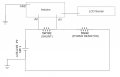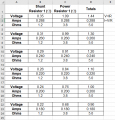# NiMh AA battery capacity tester / charger

#### chrischrischris

Joined Feb 18, 2012
313
I've been playing around trying to build a AA battery capacity checker.
Ultimately I'd like to create a discharger/charger that can handle 4 x AA and/or 4 x AAA NiMh batteries and make these for my kids - so that they'll turn away from Alkaline batteries!

I've started by using Adam Welch's thread. I've build the circuit using and Arduino Mega, Robot LCD shield, 1 ohm 5W shunt resistor, 1.2 ohm 15W power resistor to discharge through, a AA battery holder and an N channel Mosfet. The specified Mosfet is IRF3205, but I can't find one, so I've tried an IRF540, IRF 740 (which my local electronics store suggested), but the readout on the serial screen is incorrect / not working. I have some switching transistors, Darlingtons, PNP transistors, but none seems to work - I'm pulling at straw here.

On another forum, it said that I'd have to use a Mosfet with an "L" in it (something about it need to be a "Logic" mosfet. The chap said something about being able to fully open and allow 5V? Link Could this be the problem?

Battery Voltage = 1.39 Shunt Voltage = 1.50
battVolt current mAh
1.39 -0.10 -0.06

Then it goes to "complete".

Battery Capacity Checker:
/*
Battery Capacity Checker
Uses 1 Ohm power resister as shunt – Load can be any suitable resister or lamp

Required Library – LiquidCrystal.h
*/

#include <LiquidCrystal.h>
LiquidCrystal lcd(8, 9, 4, 5, 6, 7);

#define gatePin 3
#define highPin A2
#define lowPin A3

boolean finished = false;
int printStart = 0;
int interval = 5000; //Interval (ms) between measurements

float mAh = 0.0;
float shuntRes = 1.0;   // In Ohms – Shunt resistor resistance
float voltRef = 5.01;   // Reference voltage (probe your 5V pin)
float current = 0.0;
float battVolt = 0.0;
float shuntVolt = 0.0;
float battLow = 0.8;

unsigned long previousMillis = 0;
unsigned long millisPassed = 0;

void setup() {

Serial.begin(9600);
Serial.println("Battery Capacity Checker v1.1");
Serial.println("battVolt current mAh");

pinMode(gatePin, OUTPUT);
digitalWrite(gatePin, LOW);

lcd.begin(16, 2);              // start the library
lcd.clear();
lcd.setCursor(0, 0);           // go to 1st space, 1st line
lcd.print("Checking battery:");
lcd.setCursor(0, 1);           // go to 1st space, 2nd line
delay(2000);
lcd.clear();
}

void loop() {

battVolt = analogRead(highPin) * voltRef / 1024.0;
//  Serial.print("Battery Voltage = ");
//  Serial.print(battVolt);
shuntVolt = analogRead(lowPin) * voltRef / 1024.0;
//  Serial.print("  Shunt Voltage = ");
//  Serial.println(shuntVolt);

if (battVolt >= battLow && finished == false)
{
digitalWrite(gatePin, HIGH);
millisPassed = millis() - previousMillis;
current = (battVolt - shuntVolt) / shuntRes;
mAh = mAh + (current * 1000.0) * (millisPassed / 3600000.0);
previousMillis = millis();

lcd.clear();
lcd.setCursor(0, 0); // go to 1st space, 1st line
lcd.print("Discharge ");
lcd.print(battVolt);
lcd.print("v");
lcd.setCursor(0, 1); // go to 1st space, 2nd line
lcd.print("I=");
lcd.print(current);
lcd.print("a ");
lcd.print(mAh);
lcd.print("mAh");

Serial.print("Battery Voltage = ");
Serial.print(battVolt);
Serial.print("  Shunt Voltage = ");
Serial.println(shuntVolt);
Serial.print(battVolt);
Serial.print("\t");
Serial.print(current);
Serial.print("\t");
Serial.println(mAh);

delay(interval);
}
if (battVolt < battLow)
{
digitalWrite(gatePin, LOW);

finished = true;

lcd.clear();
lcd.print("Discharge");
lcd.setCursor(0, 1);
lcd.print("0mAh. Complete");
delay(interval * 2);
}
}

#### Attachments

• 2.7 KB Views: 0
Last edited:

Joined Feb 20, 2016
4,268
It may help to show the circuit you are using, and maybe a photo or two. All the more info the better.

#### chrischrischris

Joined Feb 18, 2012
313
It may help to show the circuit you are using, and maybe a photo or two. All the more info the better.
Yes, good point.

#### Attachments

Joined Feb 20, 2016
4,268
I wonder why the design has a "shunt" and "load" when one resistor could be used for both functions?
Also, the 10K resistor is shorted out, GND on both ends. It should be from GND to the FET gate I think.
I wonder if in fact you have the FET going to GND vis the 10K resistor instead of directly to GND?

#### Alec_t

Joined Sep 17, 2013
13,336
A standard IRF540/740 needs a gate-source voltage of around 10V to turn on fully. If your Arduino supply voltage is 9V-12V you could use a level-shifter circuit to convert the Arduino output pin voltage to one high enough to drive a standard MOSFET gate.

#### chrischrischris

Joined Feb 18, 2012
313
I wonder why the design has a "shunt" and "load" when one resistor could be used for both functions?
Also, the 10K resistor is shorted out, GND on both ends. It should be from GND to the FET gate I think.
I wonder if in fact you have the FET going to GND vis the 10K resistor instead of directly to GND?
Sorry, I made a mistake in my sketch (see attached). The Gnd pin of the arduino is connected to the negative of the battery being discharged via a 10K ohm resister. The signal pin D3 is going into the gate pin according to the original setup.
I've just added a 100R (well 83R as that's all I have on hand) in series with both analog inputs. I've also put in the 2 off 10nF caps from A1 to GND and A2 to GND. Same problem:

Battery Capacity Checker v1.1
battVolt current mAh
Battery Voltage = 1.39 Shunt Voltage = 1.55
1.39 -0.15 -0.09

(then it goes to complete).

I tried flipping the mosfet (gate and source) and this is what I now get:
Battery Capacity Checker v1.1
battVolt current mAh
Battery Voltage = 1.39 Shunt Voltage = 1.48
1.39 -0.09 -0.05
Battery Voltage = 4.48 Shunt Voltage = 3.15
4.48 1.33 1.80
Battery Voltage = 4.52 Shunt Voltage = 3.20
4.52 1.32 3.63
Battery Voltage = 4.49 Shunt Voltage = 3.18

4.49 1.31 5.45

I wonder if was the right way, but as Alec_t says below(above?), these mosfets need at least 10V to fully turn it on. I am only using the power source from my laptop via USB to the arduino to drive it. I think that's 5V isn't it.

#### chrischrischris

Joined Feb 18, 2012
313
A standard IRF540/740 needs a gate-source voltage of around 10V to turn on fully. If your Arduino supply voltage is 9V-12V you could use a level-shifter circuit to convert the Arduino output pin voltage to one high enough to drive a standard MOSFET gate.
Can I build a level shift circuit instead of buying another component? Where exactly would I place this in the circuit?

#### chrischrischris

Joined Feb 18, 2012
313
Could someone please check my logic below and comment.

I thought I'd go back to basics first before I select the correct Mosfet. I've re-built the circuit as follows (i.e. no digital signal from the arduino or mosfet to get the discharge started :Given my test battery is a AA, 2800mAh rechargeable, I'd like to discharge it at 1C so as to not heat it up. I'll increase this down the track. So I'm aiming at a discharge current of 280mA.

The shunt (1R2), is simply to allow me to measure the voltage drop across it, in order to work out the current and finally work out the mAh's used up - all summed up to give the total.

The power resistor I've made out of two 5W resistors in series (8.2Ω and 6.8Ω - actual values 8.3 and 7 = 3.79 in series). This value was to get close to the 280mA current (actual theoretical value is 288mA at 1.44V).

Using the tutorial on this forum , I drew up the follow spreadsheet table:I've run the circuit on my breadboard, and the figures are almost identical in real life. My question however is, looking at the spreadsheet above, as the voltage drops, the amps drop too, as it should according to ohms law. Is there a way to maintain a steady current - or is this just not neccessary (i.e. 280mA)?

PS. I do realise the above setup is dangerous - i.e. the battery voltage can drop too low. This is just a test.

#### Alec_t

Joined Sep 17, 2013
13,336
•chrischrischris

#### chrischrischris

Joined Feb 18, 2012
313
..Here's a simple level-shift circuit to demonstrate the principle
Thanks Alec. I think I understand the circuit. I assume I can use a simple switching transistor such as a 2N2222. Correct? I'm not really sure about that graph - duty cycle? Are you suggesting I pulse the NPN to pulse the mosfet at different amounts (PWM) to maintain the 280mA current discharge current?

#### Alec_t

Joined Sep 17, 2013
13,336
I assume I can use a simple switching transistor such as a 2N2222. Correct?
Correct.
Are you suggesting I pulse the NPN to pulse the mosfet
No. Just demonstrating how the output level changes with input switching by the Arduino. However, the same principle could be used for PWM.